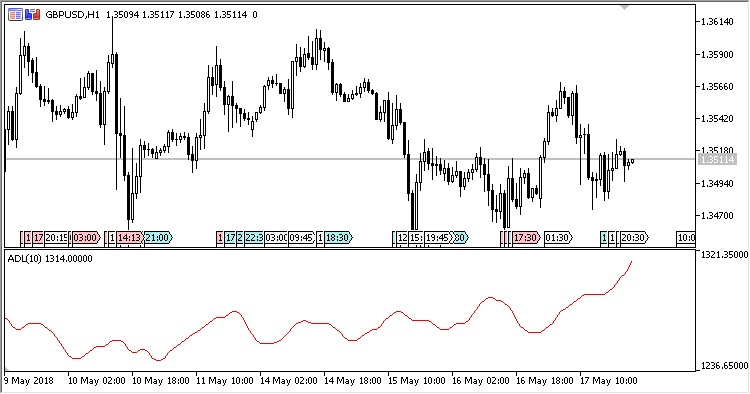# Advance_Decline_Line – indicator for MetaTrader 5

Indicator ADL (Advances/Declines Line) shows the ratio of positive and negative price increments over a specified time interval.

It has two input parameters:

• Period – calculation period;
• Use Cumulative mode – use the “cumulative” algorithm of calculations (Yes/No).

Calculations:

`ADL = (Up - Dn)`

If Use Cumulative mode = Yes, then:

`ADL = (Up - Dn) + ADL of the previous day`

where:

```Up = Up(Period)
Dn = Dn(Period)
```
• Up – number of bullish candlesticks over the period;
• Dn – number of bearish candlesticks over the period.Related Posts

Fig.1 Use Cumulative mode = Yes

Fig.2 Use Cumulative mode = No